Jan 22

# Why probability contours for the multivariate Gaussian are elliptical

Every 2D Gaussian concentrates its mass at a particular point (a “bump”), with mass falling off steadily away from its peak.  If we plot regions that have the *same* height on the bump (the same density under the PDF), it turns out they have a particular form: an ellipse.  In this post, I’ll use math to show why it is an ellipse.

Here’s an example of the kind of contour plot I’m talking about (note that this shows *many* 2D Gaussians all on one plot). The horizontal axis shows the first coordinate,, and the vertical axis shows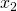. The contours illustrate values ofthat have the same probability under a particular Gaussian distribution, defined by parameters.The position of the contours is governed by the mean parameterfor each Gaussian.

The shape of the elliptical contours for each Gaussian is governed exclusively by. For example,

• the vertical (tall) ellipses (like the dark blue one) have covariance:

(1)• the forward leaning (“/”) ellipses (like the orange one) have covariance:

(2)The goal of this post is to understand *why* this elliptical structure emerges no matter what covariance matrix we specify.

## Multivariate Gaussian Math Basics

To start, we’ll remind ourselves of the basic math behind the multivariate Gaussian.

We are generating data, which is a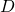-dimensional column vector.

We have two parameters:

• a mean location, which is a-dimensional column vector
• a covariance matrix, a-by-positive definite matrix

The probability density function (PDF) looks likeFor the rest of this discussion, we’ll assume that, since we’re interested in plotting in just 2 dimensions.

## Level Sets and Ellipses

We are interested in finding a setof possiblevectors such that *every* entryinhas the same value.  Furthermore, we want this set to be exhaustive, meaning no vector not in the set also has the PDF value.  This setoften called a level set of the function.  Note that each choice of a particular constantdefines a unique level set.

How can we find the level set for the Gaussian PDF? Well, we can see by the form ofthatonly influences the outcome through the term in the exponent.  So we might as well find a level setthat holds constant the transformed functionThis functionis simpler to work with, so we prefer it to the original.  The level set forgiven some constantwill be equivalent to the level set for the original PDF given some transformed constant.   It’s a nice exercise to find this transformation via algebra, but it’s not super relevant to this discussion so I’ll leave it to the reader.

## Ellipses

We’d like to show that the level set offor some constantdefines an ellipse. Recall the general form of an axis-aligned ellipse in 2D:Our goal is to show that the level set ofcan be shown to have elliptical form.  One immediate point to make is that the functionis said to have “quadratic form“, and so does the standard elliptical form.  So intuitively, we’re on the right track.  Let’s proceed.

## Change of Coordinates

The first step is to change the coordinate system.  Instead of having our system centered at the origin, (0,0), we’ll have it centered at.  This requires rewritingas, where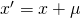.  Our new function to find level sets for is now:If we can find a level set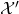for this function, we can recover the level set forjust by inverting our coordinate transform.

The next step requires some knowledge of linear algebra, so we’ll make a brief aside to brush up.

## Covariance Matrix Math Properties

Earlier, we mentioned that the covariance matrixmust be symmetric and positive definite.  This property ensures that we can always *invert* the matrix (that is,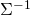exists, which is not true for all 2D matrices).  But it carries other useful properties as well.

Most importantly, ifis positive definite, then so is its inverse(see this fact sheet). This implies thatcan be decomposed into a set ofeigenvector/eigenvalue pairssuch that

• the eigenvalues are all positive reals:• the eigenvectors are all orthogonal:unless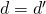So, we can write the inverse covariance matrix in terms of its eigen-decomposition.Using the following matrix definitions:

(3)Remember that because of the orthonormal requirement, we know that(the identity matrix), and this means. This fact will be useful later.

## Using this Eigendecomposition to Find Level Sets

Now, we can return to our function of interest and substitute in the decomposition:

(4)Inspecting this function closely, we can make another coordinate system transform that makes our lives simpler. If we let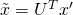, thenNote that this transformcorresponds to a single 2D rotation of the coordinate system.

Why?  Recall that any matrixis a rotation matrix if it is orthogonal and has unit determinant (see here to review).  We can show thatsatisfies both of these properties.

•(and hence) is orthogonal by definition, because its column vectors are orthonormal.
•has unit determinant:  we know, so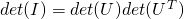. Sincewe have

(5)which implies. If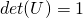, we have a valid rotation matrix. If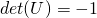,performs both rotation and reflection (e.g. flippingandin the output). Thus, the transformis a valid rotation (with a possible additional reflection).

What’s the advantage of this rotation? Becauseis just a diagonal matrix, this can be rewritten simply as a weighted sum of squares:Now, finding the level set for a constant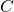in 2D reduces to solving:which can be written in elliptical form!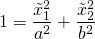whereand. Note that it’s crucial that the eigenvectorsare all positive for this analysis to hold.

We have thus accomplished our goal. Given any arbitrary covariance matrix, the level sets of the probability density function of the Gaussian will have elliptical form.